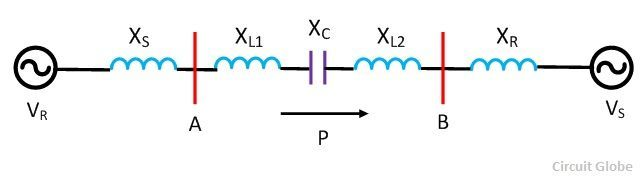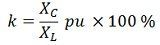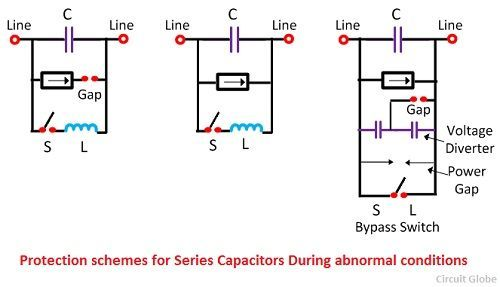# Series Compensation

Definition: Series compensation is the method of improving the system voltage by connecting a capacitor in series with the transmission line. In other words, in series compensation, reactive power is inserted in series with the transmission line for improving the impedance of the system. It improves the power transfer capability of the line. It is mostly used in extra and ultra high voltage line.

Series compensation has several advantages like it increases transmission capacity, improve system stability, control voltage regulation and ensure proper load division among parallel feeders. These advantages are discussed below.

• Increase in Power Transfer Capability – The power transfer over a line is given bywhere  P1 – power transferred per phase (W)
Vs – sending-end phase voltage (V)
Vr – receiving-end phase voltage
XL – series inductive reactance of the line
δ – phase angle between Vs and Vr

If a capacitor having capacitance reactance Xc is connected in series with the line, the reactance of the line is reduced from XL to ( XL– Xc). The power transfer is given bywhere,The factor k is known as a degree of compensation or compensation factor. Thus, per unit compensation is given by the equation percentagecompensation is given by the equationWhere XL =  total series inductive reactance of the line per phase
XC = capacitive reactance of the capacitor banck per phase
In practice , k lies between 0.4 and 0.7. For k = 0.5,Thus, the power transfer is doubled by 50 % compensation.

• Improvement in System Stability – For same power transfer and for the same value of sending and receiving end voltage, the phase angle δ in the case of the series impedance line is less that for the uncompensated line. The reduced value of δ gives higher stability.
• Load Division among Parallel Line – Series capacitors are used in transmission systems for improving the load division between parallel lines. When the new line with large power transfer capability is paralleled with an already existing line, then it is difficult to load the new line without overloading  the old line. In such case the series compensation reduces the series reactance and proper load division among parallel circuit can be done easily. Load division increases the power transfer capability of the system and reduced losses.
• Control of Voltage – In series capacitor, there is an automatic change in Var (reactive power) with the change in load current. Thus the drops in voltage levels due to sudden load variations are corrected instantly.

### Location of Series capacitor

The location of the series capacitor depends on the economic and technical consideration of the line. The series capacitor may be located at the sending end, receiving end, or at the center of the line. Sometimes they are located at two or more points along the line.

The degree of compensation and the characteristic of the line decide the location of the capacitors. Their installation at the terminal provides the facility of maintenance, but the overvoltage appearing across the terminals of the capacitors under fault conditions will over stress the capacitor.

The capacitors are installed in the intermediate switching station of comparatively long lines. The location at the center of the line also reduced the rating of the capacitor. The rating of the series capacitor is given bywhere I is the line current. Capacitor banks consist of small units connected in series, parallel, or both to get the desired voltage and Var rating.

### Protection Schemes for Series Capacitor

When the fault or overload occurs the large current will flow across the series capacitor of the line. Thus, the excessive voltage drop occurs across the transmission line. For protecting the capacitors from such abnormal voltages, spark gaps and surge diverter are connected to the capacitor terminal. A circuit breaker is also connected in parallel with it. Some of the methods of series capacitor are shown below.

###Problem associated with Series capacitor

Some of the problems associated with the series-capacitor application are given below in details

• The series compensated line produces series resonance at frequencies lower than power frequencies. This is known as sub-synchronous resonance. The sub-synchronous produces mechanical stress due to which high torsional stress occurs in the rotor shaft.The problem of sub-synchronous resonance mostly occurs during faults or switching operation. The problem of sub-synchronous  with series compensated lines is overcome by the following methods.
• By using a filter.
• By passing the series capacitor bank under resonance conditions.
• By Tripping of the generator under resonance condition.
• Series capacitors produced high recovery voltages across the breakers contact.
• If the degree of compensation and location of capacitors are not proper, the distance relays used for line protection may not function properly.
• Switching in of an unloaded transformer at the end of a series compensation of the line may produce non-linear resonance or ferro resonance. This may result in uninterrupted oscillations.The frequency of the oscillation may be suppressed by using shunt reactors across the capacitors or short circuiting the capacitors temporary.
• Lightly load synchronous motors have got a tendency to hunt.

Series capacitor produces more net voltage rise due to which more voltage drops occurs.